倒角距离变换 - CSDN

• 介绍二值图像倒角距离变换算法，帮助读者理解图像倒角距离变换分步骤实现！
图像处理之倒角距离变换图像处理中的倒角距离变换(Chamfer Distance Transform)在对象匹配识别中经常用到，算法基本上是基于3x3的窗口来生成每个像素的距离值，分为两步完成距离变换，第一步从左上角开始，从左向右、从上到下移动窗口扫描每个像素，检测在中心像素x的周围0、1、2、3四个像素，保存最小距离与位置作为结果，图示如下：第二步从底向上、从右向左，对每个像素，检测相邻像素4、5、6、7保存最小距离与位置作为结果，如图示所：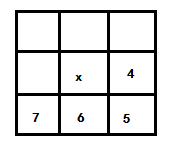完成这两步以后，得到的结果输出即为倒角距离变换的结果。完整的图像倒角距离变换代码实现可以分为如下几步：1.      对像素数组进行初始化，所有背景颜色像素点初始距离为无穷大，前景像素点距	  离为02.      开始倒角距离变换中的第一步，并保存结果3.      基于第一步结果完成倒角距离变换中的第二步4.      根据距离变换结果显示所有不同灰度值，形成图像最终结果显示如下(左边表示原图、右边表示CDT之后结果)完整的二值图像倒角距离变换的源代码如下：package com.gloomyfish.image.transform;

import java.awt.Color;
import java.awt.image.BufferedImage;
import java.util.Arrays;

import com.gloomyfish.filter.study.AbstractBufferedImageOp;

public class CDTFilter extends AbstractBufferedImageOp {
private float[] dis; // nn-distances
private int[] pos; // nn-positions, 32 bit index
private Color bakcgroundColor;

public CDTFilter(Color bgColor)
{
this.bakcgroundColor = bgColor;
}

@Override
public BufferedImage filter(BufferedImage src, BufferedImage dest) {
int width = src.getWidth();
int height = src.getHeight();

if (dest == null)
dest = createCompatibleDestImage(src, null);

int[] inPixels = new int[width * height];
pos = new int[width * height];
dis = new float[width * height];
src.getRGB(0, 0, width, height, inPixels, 0, width);
// 随机生成距离变换点
int index = 0;
Arrays.fill(dis, Float.MAX_VALUE);
int numOfFC = 0;
for (int row = 0; row < height; row++) {
for (int col = 0; col < width; col++) {
index = row * width + col;
if (inPixels[index] != bakcgroundColor.getRGB()) {
dis[index] = 0;
pos[index] = index;
numOfFC++;
}
}
}
final float d1 = 1;
final float d2 = (float) Math.sqrt(d1 * d1 + d1 * d1);
System.out.println(numOfFC);
float nd, nd_tmp;
int i, in, cols, rows, nearestPixel;

// 1 2 3
// 0 i 4
// 7 6 5
// first pass: forward -> L->R, T-B
for (rows = 1; rows < height - 1; rows++) {
for (cols = 1; cols < width - 1; cols++) {
i = rows * width + cols;

nd = dis[i];
nearestPixel = pos[i];
if (nd != 0) { // skip background pixels
in = i;

in += -1; // 0
if ((nd_tmp = d1 + dis[in]) < nd) {
nd = nd_tmp;
nearestPixel = pos[in];
}

in += -width; // 1
if ((nd_tmp = d2 + dis[in]) < nd) {
nd = nd_tmp;
nearestPixel = pos[in];
}

in += +1; // 2
if ((nd_tmp = d1 + dis[in]) < nd) {
nd = nd_tmp;
nearestPixel = pos[in];
}

in += +1; // 3
if ((nd_tmp = d2 + dis[in]) < nd) {
nd = nd_tmp;
nearestPixel = pos[in];
}

dis[i] = nd;
pos[i] = nearestPixel;
}
}
}

// second pass: backwards -> R->L, B-T
// exactly same as first pass, just in the reverse direction
for (rows = height - 2; rows >= 1; rows--) {
for (cols = width - 2; cols >= 1; cols--) {
i = rows * width + cols;

nd = dis[i];
nearestPixel = pos[i];
if (nd != 0) {
in = i;

in += +1; // 4
if ((nd_tmp = d1 + dis[in]) < nd) {
nd = nd_tmp;
nearestPixel = pos[in];
}

in += +width; // 5
if ((nd_tmp = d2 + dis[in]) < nd) {
nd = nd_tmp;
nearestPixel = pos[in];
}

in += -1; // 6
if ((nd_tmp = d1 + dis[in]) < nd) {
nd = nd_tmp;
nearestPixel = pos[in];
}

in += -1; // 7
if ((nd_tmp = d2 + dis[in]) < nd) {
nd = nd_tmp;
nearestPixel = pos[in];
}

dis[i] = nd;
pos[i] = nearestPixel;

}
}
}

for (int row = 0; row < height; row++) {
for (int col = 0; col < width; col++) {
index = row * width + col;
if (Float.MAX_VALUE != dis[index]) {
int gray = clamp((int) (dis[index]));
inPixels[index] = (255 << 24) | (gray << 16) | (gray << 8)
| gray;
}
}
}
setRGB(dest, 0, 0, width, height, inPixels);
return dest;
}

private int clamp(int i) {
return i > 255 ? 255 : (i < 0 ? 0 : i);
}

}转载请注明出处！！
展开全文图像处理 JAVA 算法
• ## 倒角距离变换

千次阅读 2017-08-04 07:38:26
这个名词两年前看文章就看到了，可是那时候还不知道什么叫博士，不知道要挣钱养家，于是乎态度就是管他是什么，知道有这么个东西就OK了。两年里，这个词我看了不下5遍了，始终也没想搞明白。终于，最近才对此有些...

这个名词两年前看文章就看到了，可是那时候还不知道什么叫博士，不知道要挣钱养家，于是乎态度就是管他是什么，知道有这么个东西就OK了。两年里，这个词我看了不下5遍了，始终也没想搞明白。终于，最近才对此有些兴趣。可是查这个方法的时候，发现文章最新的也是在上个世纪80年代的，在文献里发现作者都是上一代甚至上两代的“圣斗士”。但是花了好大的力气，才看明白这个方法。发现关于这个方法的很多概念都不是很清晰的，那个时候，计算机视觉和图像处理还不是一个热门的话题吧。发现了很多概念，chamfer
metrics(chamfer distance), distance transform, sequential distance transform。最经典的一篇文献就是88年PAMI上的hierachical chamfer matching: a parametric edge matching algorithm。看了这个文章，又查了一些网页诸如http://www.spatialanalysisonline.com/OUTPUT/html/Distancetransforms.html。才明白啥是chamfer，chamfer本意是砍树出现很多锯齿的斜面，这里所谓chamfer就是在离散的数字图像上近似欧式距离时候，也会产生很多有锯齿的斜面，所以叫做chamfer
metric。而现在所谓的chamfer matching都是指最早的sequential distance transform。说白了这个方法就是采用distance transform的方法是如何鲁棒的匹配一个模板。而且matlab中还有一个函数bwdist()专门完成这个功能，我运行了一下，一开始不是很理解，我以为不是一个函数，后来就自己遍了一段代码，结果发现结果是一样的。后来仔细分析一下，才感觉，就应该像bwdist()那样的运行效果。不过自己编写了一遍，还能找点感觉。暂且贴段代码吧。

#include "chamferDT.h"
#include "assert.h"
#define INFINITE_NUM 1000000
//sequential distance transform using (4,3) distance
void chamferDT(IplImage*edge,      //the edge images, single channel images
IplImage*dt,           //the
distance transform images
float threshold){
assert(edge!=NULL);
assert(dt!=NULL);
int width = edge->width;
int height = edge->height;
int widthStep = edge->widthStep;

cvCopyImage(edge,dt);

BYTE *imdata =(BYTE*) dt->imageData;

for(int i=0; i<height; i++)
for(int j=0; j<width; j++)
imdata[i*widthStep+j] = (imdata[i*widthStep+j]<threshold ? INFINITE_NUM : 0);
//forward process
for(int
i=1; i<height; i++)
for(int j=1; j<width-1; j++){
imdata[i*widthStep+j] = min(min(min(min(imdata[(i-1)*widthStep+j-1]+4,imdata[(i-1)*widthStep+j]+3),
imdata[(i-1)*widthStep+(j+1)]+4),
imdata[i*widthStep+j-1]+3),
imdata[i*widthStep+j]);
}

//backward process
for(int i=height-2; i>=0; i--)
for(int j=width-2; j>=1; j-- ){
imdata[i*widthStep+j]
= min(min(min(min(imdata[i*widthStep+j],imdata[i*widthStep+j+1]+3),
imdata[(i+1)*widthStep+j-1]+4),
imdata[(i+1)*widthStep+j]+3),
imdata[(i+1)*widthStep+j+1]+4);
}

for(int i=0; i<height; i++)
for(int j=0; j<width; j++)
imdata[i*widthStep+j] =(imdata[i*widthStep+j]*6 > 255 ? 255
: imdata[i*widthStep+j]*6) ;
}

转载自http://blog.sina.com.cn/s/blog_78f6d29e0100qc3x.html

展开全文• 倒角距离变换(Chamfer Distance Transform) 图a,b为距离变换模板，设其值分别为a[1-5],b[5-9]。 图c为待处理的二维二值化图像，其中1代表目标区域，0代表背景区域。设其值为c[m][n]。 目标是求出目标区域中的每一个...
倒角距离变换(Chamfer Distance Transform)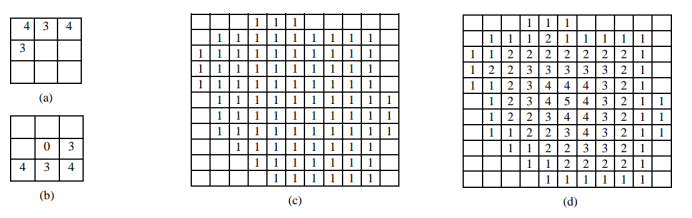图a,b为距离变换模板，设其值分别为a[1-5],b[5-9]。
图c为待处理的二维二值化图像，其中1代表目标区域，0代表背景区域。设其值为c[m][n]。
目标是求出目标区域中的每一个点距离背景区域的最小距离，即图d。
倒角距离变化算法思路如下：
(1)首先对原始二值矩阵进行放大得到c2，整体乘以一个膨胀因子p。p的大小会决定能算出的最大距离，即求出的最终距离矩阵中的数值上界，p越大距离越大。
(2)使用距离变换模板图a对二值化图像c2从左到右、从上到下的进行移动遍历。如果该点为0则跳过，为1则使用模板a对应该点，计算该点的a[1-5]所对应的每一个点的像素值与a中每一个点的模板值之和。
如果该点为矩阵的四周上的点，即模板上部分点超出了原始矩阵的边界，则设置这些点的像素值为极大值1*p。不过为了优化算法效率，实际计算时可以仅对矩阵的非边界区域进行操作，即前向时不计算第1行，第1列和最后1列。这样做的最终效果是：只有地图的左右两列未被计算，并不影响我们在局部路径规划中的使用。
最终该点保留的值为这5个和值的最小值，得到矩阵f1具体过程以下图为例：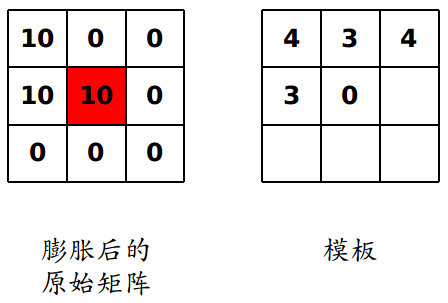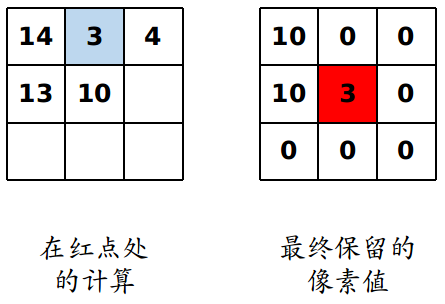(3)使用模板b对前向遍历的结果矩阵f1从右到左、从下到上的进行移动遍历。操作与上一步相同，得到矩阵f2。同上，不计算矩阵的最后1行，第1列和最后1列。
(4)f2即为最终矩阵，除以3之后形成图d，效果图如下：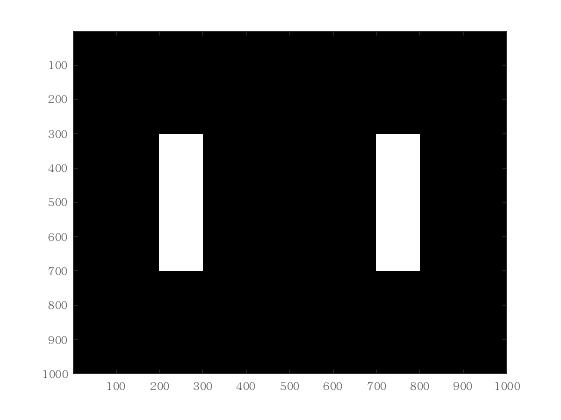原始二值地图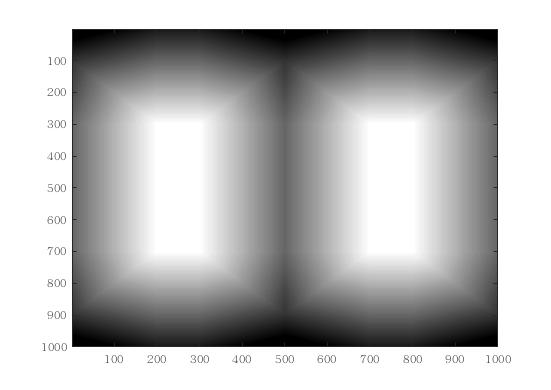距离地图
%对二值地图进行倒角距离变换，输出一张距离地图。
inf_factor=1200;%膨胀因子，代表像素值的上线，极大值
raw_a=zeros(1000,1000);
for i=300:700
for j=200:300
raw_a(i,j)=1;
end
end
for i=300:700
for j=700:800
raw_a(i,j)=1;
end
end
raw_a=double(~raw_a)*inf_factor;

figure('NumberTitle', 'off', 'Name', '原始二值地图');
imagesc(raw_a);
colormap(flipud(gray));

h=size(raw_a,1);
w=size(raw_a,2);
a_transpro=raw_a;

tic
for i=2:h%前向遍历过程，从上到下、从左到右
for j=2:w-1
tmp=a_transpro(i,j);%初值取目标像素点初始值
if a_transpro(i,j)%只操作前景点，即为1的点
tmp=min(a_transpro(i-1,j-1)+4,tmp);
tmp=min(a_transpro(i-1,j)+3,tmp);
tmp=min(a_transpro(i-1,j+1)+4,tmp);
tmp=min(a_transpro(i,j-1)+3,tmp);
a_transpro(i,j)=tmp;%最终保留几个和值的最小值作为该点距离值
end
end
end

for i=h-1:-1:1%从下到上、从右到左
for j=w-1:-1:2
tmp=a_transpro(i,j);
if a_transpro(i,j)
tmp=min(a_transpro(i+1,j+1)+4,tmp);
tmp=min(a_transpro(i+1,j)+3,tmp);
tmp=min(a_transpro(i+1,j-1)+4,tmp);
tmp=min(a_transpro(i,j+1)+3,tmp);
a_transpro(i,j)=tmp;
end
end
end

a_final=a_transpro/3;%遍历结束后除以3作为最终结果
t=toc

figure('NumberTitle', 'off', 'Name', '距离地图');
imagesc(a_final);
colormap(flipud(gray));




展开全文• 主要为大家详细介绍了java图像处理之倒角距离变换的相关资料，具有一定的参考价值，感兴趣的小伙伴们可以参考一下
• ## 倒角距离匹配

千次阅读 2017-08-04 09:34:09
Chamfer Matching Chamfer matching是一种进行图像匹配的方法，最早见于(Barrow,1977)。 构造距离变换 方法的名字chamfer指的是...对于一个有特征点(*)和非特征点(-)组成的二值图像，距离变换就是求得每一个点到最近
Chamfer Matching
Chamfer matching是一种进行图像匹配的方法，最早见于(Barrow,1977)。
构造距离变换
方法的名字chamfer指的是一个求取距离变换(DT, distance transform, distance function)的过程。 距离变化的一个例子如下。对于一个有特征点(*)和非特征点(-)组成的二值图像，距离变换就是求得每一个点到最近特征点的距离。早期文献中用到的特征点都是边缘点。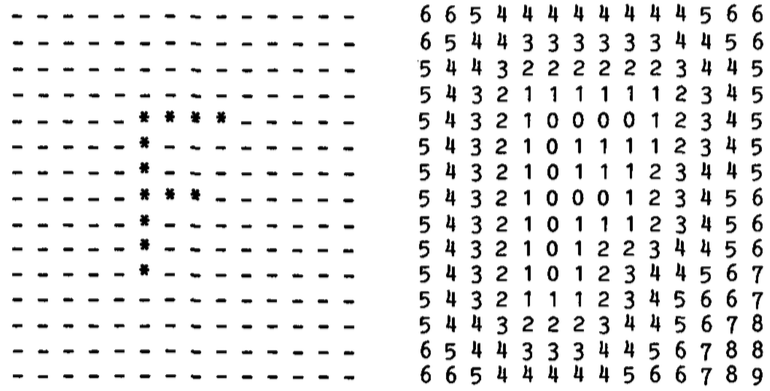匹配过程
对两幅图像进行匹配的过程是这样的：为其中一幅计算DT，然后将另一幅的特征点叠加在DT上，计算特征点对应的DT值的均值。这是原始的论文给出的方法。下图显示了将待匹配的曲线叠加在DT上。曲线和图像的距离就可以通过叠加点上DT值的某种均值来计算。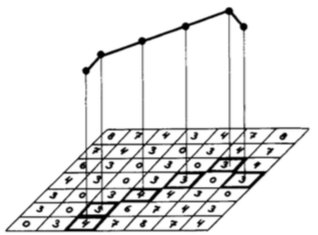后来的文章(Borgefors,1988)认为，采用均值不是最佳的策略，为了避免假的匹配，一种更好地方法是计算root mean square (r.m.s)：
131n∑i=1nv2i−−−−−−−√

匹配的图像可以在DT上平移、旋转、缩放，因此，匹配的过程就是寻找到一个最优的位置。由于存在众多的局部极小点，这个优化过程是不能够简单的采用某些梯度下降的方法得到的。
Hierarchical Chamfer Matching
文章(Borgefors,1988)给出了一种优化的过程。 从文章的题目可知，第一步是构建图像金字塔， 构建的方法是对原始边缘图进行比例为2的降采样。 降采样的方法是在2x2的小块上进行OR，即或运算。 这一步得到的叫做边缘金字塔。对边缘金字塔进行距离变换，得到的叫做距离金字塔。
用来构建距离金字塔的图像叫做pre-distance image。带匹配的图像叫做pre-polygon image。 在将polygon叠加在DT上之前，可以进行一些变换，这些变换用参数表示，比如平移可以用一对参数(x,y)表示。优化的过程就是按照一定的步长(dx,dy)调整参数，在参数空间的邻近点上寻找局部最优值。比如，调整x，就可以比较f(x+dx),f(x),f(x-dx)获取x的优化方向，如此迭代直至找到局部最优。当参数维度较大时，同时比较参数空间各个维度上的邻近点会导致严重的性能问题。因此，文章采取住个参数调整的方法。
优化的过程从一个较粗的尺度开始，以若干个不同的参数值为起点，分别独立的进行优化。在得到一组局部最优点后，根据一些原则拒绝掉其中的一部分。剩余的局部最优点再次作为起点，在下一个尺度层次上进行优化。
参考文献
Barrow, H. G., Tenenbaum, J. M., Bolles, R. C., & Wolf, H. C. (1977). Parametric correspondence and chamfer matching: Two new techniques for image matching.Borgefors, G. (1986). Distance transformations in digital images. Computer vision, graphics, and image processing, 34(3), 344-371.Borgefors, G. (1988). Hierarchical chamfer matching: A parametric edge matching algorithm. IEEE T-PAMI, 10(6), 849-865.
展开全文• 距离变换于1966年被学者首次提出,目前已被广泛应用于图像分析、计算机视觉、模式识别等领域，人们利用它来实现目标细化、骨架提取、形状插值及匹配、粘连物体的分离等。距离变换是针对二值图像的一种变换。在二维...距离变换
• 定义：一种对于图像的距离变换（distance transform），常用于shaped based object detection。对于一个有特征点和非特征点的二值图像，此距离变换就是求解每一个点到最近特征点的距离。 距离变换就是将一幅表示目标...
• 原文地址 [研究内容] 二值图像距离变换 [正文] 二值图像距离变换的概念由Rosenfeld和Pfaltz于1966年在论文中提出，目前广泛应用于...距离变换按照距离的类型可以分为欧式距离变换(Eudlidean Distance Transfrom)
• ## opencv 距离变换

千次阅读 2016-11-05 23:48:06
二值图像距离变换的概念由Rosenfeld和Pfaltz于1966年在论文中提出，目前广泛应用于计算机图形学，目标识别及GIS空间分析等领域，其主要思想是通过表识空间点(目标点与背景点)距离的过程，就是通过使用两遍扫描光栅...opencv 算法
• 之前一直以为距离变换的意义就在于骨架抽取，看到一篇论文用距离变换的方式来实现匹配，配合倒角距离变换（Chamfer Distance Transform）可以达到快速匹配的效果
• 很多博客都在讲倒角距离变换（chamfer distance transform），看完之后，我对倒角距离仍然是一片雾水。因此，在这篇文章论述一下我对倒角距离的理解。 前言：距离变换 距离变换的主要目的是通过识别目标点与背景点，...计算机视觉 人工智能
• 二值图距离变换有多种方法，在此只简述Chamfer倒角距离变换法。 距离变换算法过程如下： 首先给出变化操作区域（也可以称为模板） 1 2   4 X   7         3   X
• 一种对于图像的距离变换（distance transform），常用于shaped based object detection。对于一个有特征点和非特征点的二值图像，此距离变换就是求解每一个点到最近特征点的距离。 Chamfer Distance 匹配过程...
• 1、象素间各种距离的定义及计算 我们知道，构成一幅数字图像最基本的元素是一个一个的像素点，也就是像素。理解像素间的一些基本关系是我们以后进行图形图形处理的基础和关键。如相邻像素（像素的邻域），像素的...
...